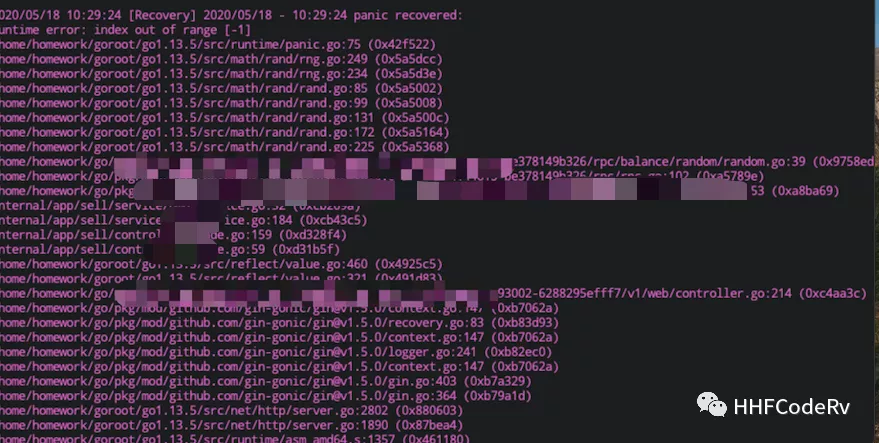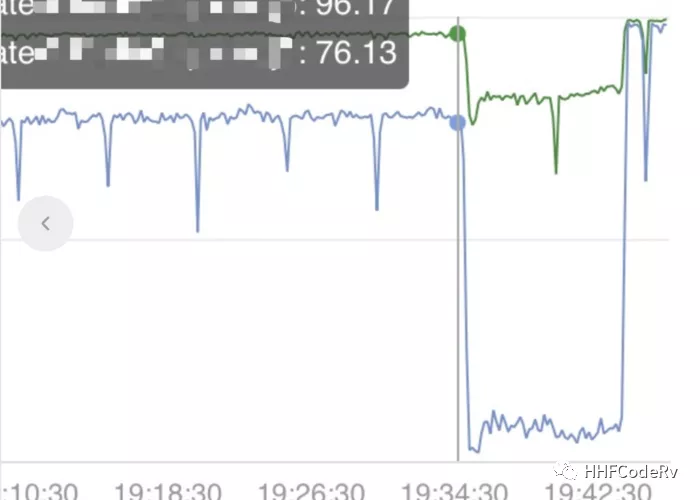Go Rand is a feature often used in development, but there are some pitfalls in it. This article will completely break down Go math/rand and make it easy for you to use Go Rand.

First of all, a question: Do you think rand will panic ?## Source Code Analysis

The math/rand source code is actually quite simple, with just two important functions.

 `````` 1 2 3 4 5 6 7 8 9 10 11 12 13 14 15 16 17 18 19 20 `````` ``````func (rng *rngSource) Seed(seed int64) { rng.tap = 0 rng.feed = rngLen - rngTap //... x := int32(seed) for i := -20; i < rngLen; i++ { x = seedrand(x) if i >= 0 { var u int64 u = int64(x) << 40 x = seedrand(x) u ^= int64(x) << 20 x = seedrand(x) u ^= int64(x) u ^= rngCooked[i] rng.vec[i] = u } } } ``````

This function is setting the seed, which is actually setting the value of each position of rng.vec. The size of rng.vec is 607.

 `````` 1 2 3 4 5 6 7 8 9 10 11 12 13 14 15 `````` ``````func (rng *rngSource) Uint64() uint64 { rng.tap-- if rng.tap < 0 { rng.tap += rngLen } rng.feed-- if rng.feed < 0 { rng.feed += rngLen } x := rng.vec[rng.feed] + rng.vec[rng.tap] rng.vec[rng.feed] = x return uint64(x) } ``````

This is the function we end up calling when we use other functions like Intn(), Int31n(), etc. You can see that each call is the result of adding two values from rng.vec using the rng.feed rng.tap. The result is also put back into rng.vec.

Note here that when using rngSource with rng.go, since rng.vec sets the value of rng.vec at the same time as the random number, there will be data competition when multiple goroutines are called at the same time. math/rand solves this by locking sync.Mutex when calling rngSource.

 ``````1 2 3 4 5 6 `````` ``````func (r *lockedSource) Uint64() (n uint64) { r.lk.Lock() n = r.src.Uint64() r.lk.Unlock() return } ``````

We can also use `rand.Seed()` , `rand.Intn(100)` directly, because math/rand initializes a globalRand variable.

 ``````1 2 3 4 5 `````` ``````var globalRand = New(&lockedSource{src: NewSource(1).(*rngSource)}) func Seed(seed int64) { globalRand.Seed(seed) } func Uint32() uint32 { return globalRand.Uint32() } ``````

Note that since the call to rngSource is locked, using `rand.Int32()` directly will result in global goroutine lock contention, so in high concurrency scenarios, when your program’s performance is stuck here, you need to consider using `New(&lockedSource{src: NewSource(1). (*rngSource)})` to generate separate rands for different modules. However, based on current practice, using globalRand locks is not as competitive as we might think. There is a pitfall in using New to generate a new rand, which is how the panic in the opening post was created, as we will see later.

## Seed What exactly is the role of seeds?

 ``````1 2 3 4 5 6 7 `````` ``````func main() { for i := 0; i < 10; i++ { fmt.Printf("current:%d\n", time.Now().Unix()) rand.Seed(time.Now().Unix()) fmt.Println(rand.Intn(100)) } } ``````

Results:

 ``````1 2 3 4 5 6 7 `````` ``````current:1613814632 65 current:1613814632 65 current:1613814632 65 ... ``````

This example leads to the conclusion that the same seed will give the same result every time it is run. Why is that?

When you use math/rand, you must set the seed by calling rand.Seed, which actually sets the corresponding value for the 607 slots in rng.vec. Seed will call a seedrand function to calculate the value of the corresponding slot.

 `````` 1 2 3 4 5 6 7 8 9 10 11 12 13 14 15 `````` ``````func seedrand(x int32) int32 { const ( A = 48271 Q = 44488 R = 3399 ) hi := x / Q lo := x % Q x = A*lo - R*hi if x < 0 { x += int32max } return x } ``````

The results of this function are not random, but are actually calculated according to seed. In addition, this function is not just written, but has a mathematical proof.

This means that the same seed is set to the same value in rng.vec, and the same value is retrieved by Intn.

## The traps I encountered

### 1. rand panic

The screenshot at the beginning of the article is a panic that occurred one day during the development of the project using the underlying library wrapped by someone else. The approximate code implementation is as follows.

 `````` 1 2 3 4 5 6 7 8 9 10 11 12 13 14 `````` ``````// random.go var ( rrRand = rand.New(rand.NewSource(time.Now().Unix())) ) type Random struct{} func (r *Random) Balance(sf *service.Service) ([]string, error) { // .. 通过服务发现获取到一堆ip+port, 然后随机拿到其中的一些ip和port出来 randIndexes := rrRand.Perm(randMax) // 返回这些ip 和port } ``````

This Random will be called concurrently, and since rrRand is not concurrently safe, it will occasionally panic when calling rrRand.

When using math/rand, some people use math.Intn() to initialize a new rand with rand.New, because they are worried about lock competition, but note that the rand initialized by rand.New is not concurrency-safe.

The fix: replace rrRand with globalRand, which has little effect on global locking in online high concurrency scenarios.

### 2. always load to the same machineIt is also an underlying rpc library that uses random traffic distribution, and after running online for a while, the traffic is routed to one machine, causing the service to go down. The approximate implementation code is as follows.

 `````` 1 2 3 4 5 6 7 8 9 10 11 12 13 14 15 16 17 18 19 20 21 22 23 24 25 26 27 `````` ``````func Call(ctx *gin.Context, method string, service string, data map[string]interface{}) (buf []byte, err error) { ins, err := ral.GetInstance(ctx, ral.TYPE_HTTP, service) if err != nil { // 错误处理 } defer ins.Release() if b, e := ins.Request(ctx, method, data, head); e == nil { // 错误处理 } // 其他逻辑, 重试等等 } func GetInstance(ctx *gin.Context, modType string, name string) (*Instance, error) { // 其他逻辑.. switch res.Strategy { case WITH_RANDOM: if res.rand == nil { res.rand = rand.New(rand.NewSource(time.Now().Unix())) } which = res.rand.Intn(res.count) case 其他负载均衡查了 } // 返回其中一个ip和port } ``````

The reason for the problem: It can be seen that each request comes with an ip and port using GetInstance, and if we use the Random method of traffic load balancing, each time we reinitialize a rand. We already know that when the same seed is set, the result is the same for each run. When the instant traffic is too large, the concurrent request GetInstance, because the value of time.Now().Unix() is the same at that moment, this will result in getting the same random number, so the last ip, port are the same, the traffic is distributed to this machine.

Fix: Change it to globalRand.

## rand future expectations

Basically, you can see that in order to prevent global lock competition, when using math/rand, the first thing that comes to mind is custom rand, but it’s easy to get into some kind of trouble.

Why does math/rand need to be locked?

We all know that math/rand is pseudo-random, but after setting the seed, the value of rng.vec array is basically determined, which is obviously not random anymore. .vec, so it is necessary to lock rng.vec to protect it.

Using rand.Intn() does have a global lock competition problem, I wonder if math/rand will be optimized in the future.